# PSEB 3rd Class Maths Solutions Chapter 10 Data Handling

Punjab State Board PSEB 3rd Class Maths Book Solutions Chapter 10 Data Handling Textbook Exercise Questions and Answers.

## PSEB Solutions for Class 3 Maths Chapter 10 Data Handling

PAGE 197:

Question 1.
The following pictograph shows cartoon programs liked by students of the school.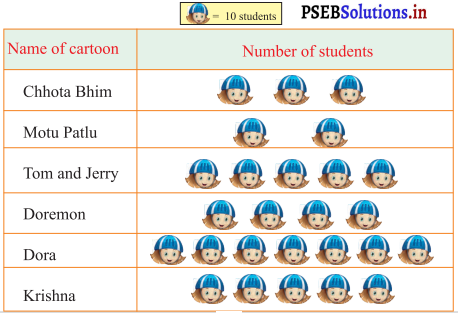PAGE 198:

Answer the questions on the basis of above pictograph:

(i) Which cartoon is most popular among students ?
Solution.
Dora.

(ii) Which cartoon is least popular among students ?
Solution.
Motu Patlu.

(iii) How many student like the cartoon of Doremon?
Solution.
4 × 10 = 40.

(iv) Which of the two cartoons are equally popular among students?
Solution.
Tom and Jerry and Krishna.

(v) How many students like Chhota Bheem and Motu Patlu ?
Solution.
(3 + 2) × 10 = 5 × 10 = 50.

(vi) Is there any other way to represent the above data ?
Solution.
Yes.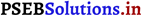Question 2.
Look at the pictograph given below and answer the questions : ||B| = 3 runs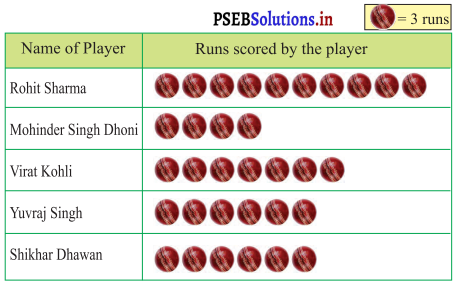(i) Who scored maximum number of runs and how many ?
Solution.
Rohit Sharma scored maximum 10 × 3 = 30 runs.

(ii)
Who scored minimum number of runs and how many ?
Solution.
Mahinder Singh Dhoni scored minimum 4 × 3 = 12 runs.

(ii)
Which two players scored same number of runs and how many ?
Solution.
Yuvraj Singh and Shikhar Dhawan both scored 6 × 3 = 18 runs each.

(iv) Total runs scored by Shikhar Dhawan and Virat Kohli ?
Solution.
Shikhar Dhawan and Virat Kohli both scored (6 + 7) × 3 = 39 runs.Question 3.
The following table shows the marks obtained by a III class student Gurjot in her exams :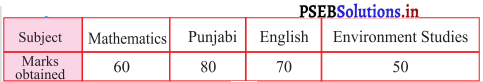Solution.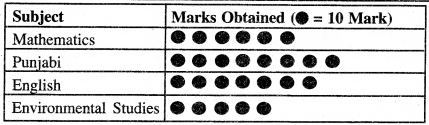Question 4.
In a school, five classes have been given the responsibility to take care of 5 flower beds. The following table shows the number of flowers in each bed, alloted to the different classes: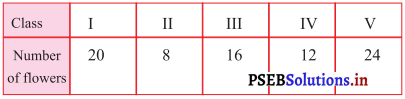Represent the above information with the help of a pictograph:
Assume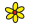= 2 Flowers
Solution.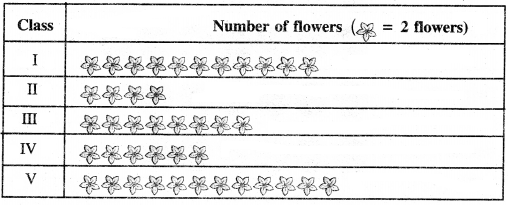PAGE 200:
Let’s learn:

Question 1.
The different figure shapes of maths kit are shown in the following box: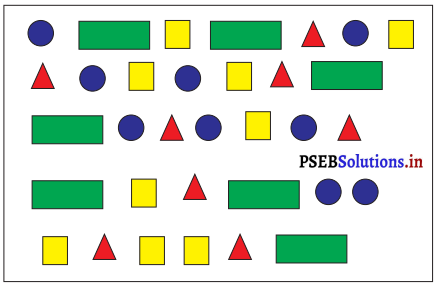1. Represent the above data by using tally marks:
Solution.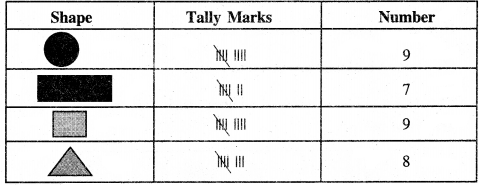Question 2.
Today is Manjit Singh’s birthday. He is the student of 3rd class. His classmates have decorated a wall of the classroom with some colourful balloons.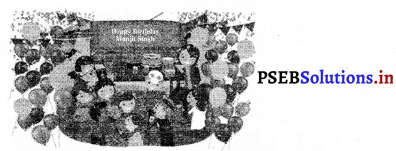Represent the information given at previous page with the help of tally marks.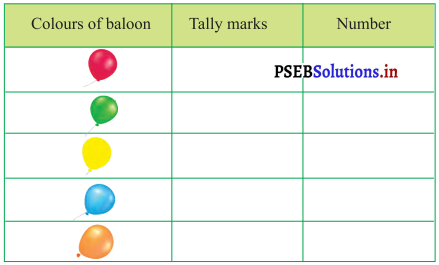Solution.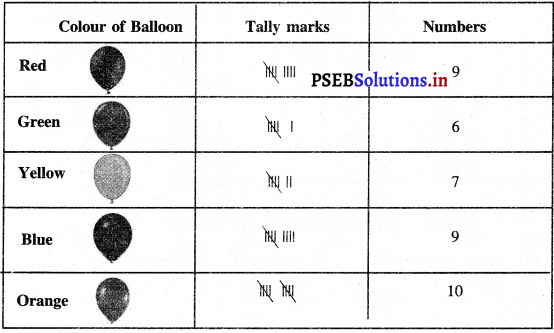Find:
(i) Which colour has maximum number of balloons ?
Solution.
Orange.

(ii) How many yellow balloons are there ?
Solution.
Seven.

(iii) How many balloons are there in total ?
Solution.
41.

Question 3.
Third class students were asked about their favourite fruits. Their choices are given below: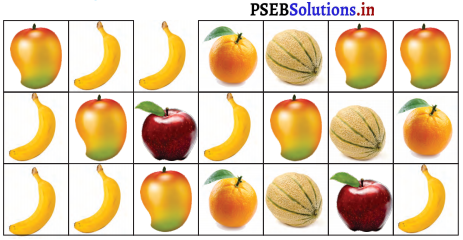PAGE 203:

Represent the information given at previous page with the help of tally marks :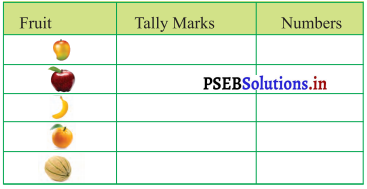Solution.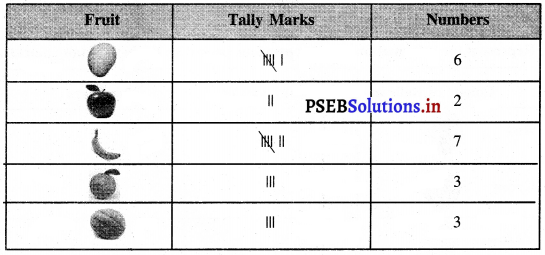Find :
(i) Which fruit is the choice of maximum students?
Solution.
Banana.

(ii) Which fruit is the choice of least number of students?
Solution.
Apple.

(iii) How many students opt for Banana?
Solution.
7.

(iv) How many students like Apples?
Solution.
2.

Question 4.
The following table shows the favourite games of Third class students: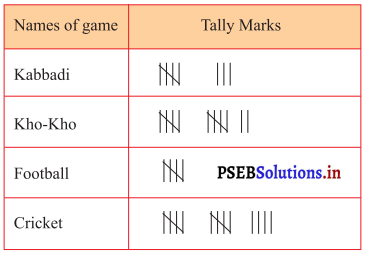PAGE 204:

(i) Which is the most favourite game of students ?
Solution.
Cricket.

(ii) Which is the least favourite game of students ?
Solution.
Football.

(iii) How many students like to play Kho-Kho ?
Solution.
12.

(iv) How many students in total like to play Football and Cricket ?
Solution.
19.

(v) How many students are studying in class in ?
Solution.
39.Question 5.
The table given below shows the favourite colours of 3rd class students: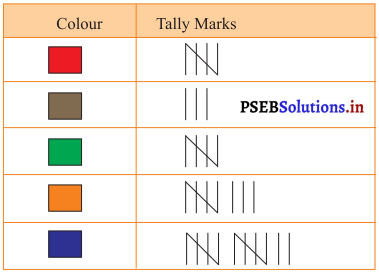(i) Which is the most favourite colour of the students ?
Solution.
Blue.

(ii) Which is the least favourite colour of the students?
Solution.
Brown.

(iii) How many students like red colour ?
Solution.
5.

(iv) Which colours are equally liked by the students?
Solution.
Red and Green.

(v) How many students study in class HI?
Solution.
33.Worksheet:

Question 1.
If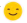= 5 students then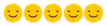= ____ students.
Solution.
25

Question 2.
In a school’s annual day function four students of class III made bouquets that are shown in the pictograph as below.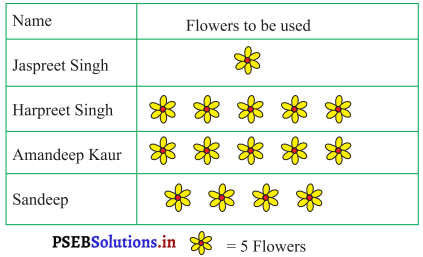(i) Jaspreet Singh used one flower to make a bouquet. (✓ Or ✗)
Solution.
✗.

(ii) Sandeep used 20 flowers to make a bouquet. (✓ Or ✗)
Solution.
✓.

(iii) Sandeep used maximum number of flowers to make a bouquet. (✓ Or ✗)
Solution.
✗.(iv) Which of the student used 5 flowers to make a bouquet?
(a) Jaspreet Singh
(b) Harpreet Singh
(c) Amandeep Kaur
(d) Sandeep.
Solution.
(a) Jaspreet Singh.

(v) All students used _______ flowers to make a bouquet.
Solution.
15 × 5 = 75.

(vi) Which of the two students used same number of flowers to make bouquets ?
(a) Jaspreet Singh and Harpreet Singh
(b) Harpreet Singh and Amandeep Kaur
(c) Amandeep Kaur and Sandeep
(d) Harpreet Singh and Sandeep.
Solution.
(b) Harpreet Singh and Amandeep Kaur.(vii) Which tally marks is used to show the flowers used by Amandeep Kaur ?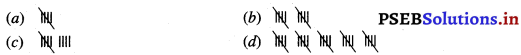Solution.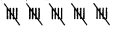(viii) Which student used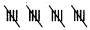for the number of flowers show n in the tally marks?
(a) Amandeep Kaur
(b) Jaspreet Singh
(c) Sandeep
(d) Harpreet Singh
Solution.
(c) Sandeep.(ix) In the above table stands for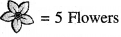(✓ Or ✗)
Solution.

Multiple Choice Questions:

(i) The information about the children going to different place during summer, vacations is shown in the following table. After studying the table answer the following questions.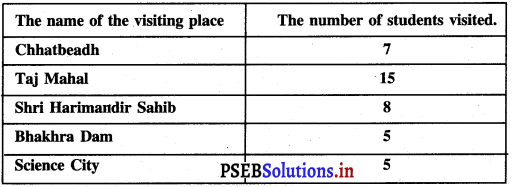Question 1.
What is the number of children going to Harmandir Sahib ?
(a) 48
(b) 15
(c) 8
(d) 7
Solution.
(c) 8.

Question 2.
The number of Children going to Chhatbeadh is ______ than going to Bhakhra Dam.
(a) Less
(b) More
(c) Equal
(d) None of these.
Solution.
(b) More.Question 3.
Which number is ‘shown by the telly mark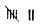?
Solution.
7.